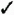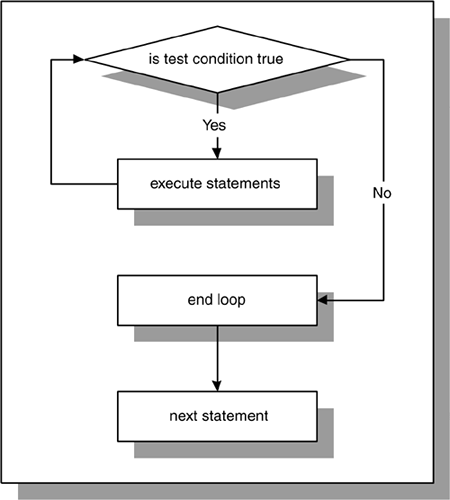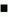# Lab 8.2 WHILE Loops

## Lab Objectives

After this Lab, you will be able to:Use WHILE Loops

A WHILE loop has the following structure:

` `
` WHILE  CONDITION  LOOP    STATEMENT 1;    STATEMENT 2;    ...    STATEMENT N; END LOOP; `

The reserved word WHILE marks the beginning of a loop construct. The word CONDITION is the test condition of the loop that evaluates to TRUE or FALSE. The result of this evaluation determines whether the loop is executed. Statements 1 through N are a sequence of statements that is executed repeatedly. The END LOOP is a reserved phrase that indicates the end of the loop construct.

This flow of the logic is illustrated in Figure 8.3.

##### Figure 8.3. WHILE LoopFigure 8.3 shows that the test condition is evaluated prior to each iteration of the loop. If the test condition evaluates to TRUE, the sequence of statements is executed, and control is passed to the top of the loop for the next evaluation of the test condition. If the test condition evaluates to FALSE, the loop is terminated , and control is passed to the next executable statement following the loop.

As mentioned earlier, before the body of the loop can be executed, the test condition must be evaluated. The decision as to whether to execute the statements in the body of the loop is made prior to entering the loop. As a result, the loop will not be executed at all if the test condition yields FALSE.FOR EXAMPLE

` `
` DECLARE    v_counter NUMBER := 5; BEGIN    WHILE v_counter < 5 LOOP       DBMS_OUTPUT.PUT_LINE ('v_counter = 'v_counter);       -- decrement the value of v_counter by one       v_counter := v_counter - 1;   END LOOP; END; `

In this example, the body of the loop is not executed at all because the test condition of the loop evaluates to FALSE.

While the test condition of the loop must evaluate to TRUE at least once for the statements in the loop to execute, it is important to insure that the test condition will eventually evaluate to FALSE, as well. Otherwise, the WHILE loop will execute continually.FOR EXAMPLE

` `
` DECLARE    v_counter NUMBER := 1; BEGIN    WHILE v_counter < 5 LOOP       DBMS_OUTPUT.PUT_LINE('v_counter = 'v_counter);       -- decrement the value of v_counter by one       v_counter := v_counter - 1;   END LOOP; END; `

This is an example of an infinite WHILE loop. The test condition always evaluates to TRUE, because the value of v_counter is decremented by 1 and is always less than 5.It is important to note that Boolean expressions can also be used to determine when the loop should terminate. ` ` ` DECLARE v_test BOOLEAN := TRUE; BEGIN WHILE v_test LOOP STATEMENTS; IF TEST_CONDITION THEN v_test := FALSE; END IF; END LOOP; END; ` When using a Boolean expression as a test condition of a loop, you must make sure that a different value is eventually assigned to the Boolean variable in order to exit the loop. Otherwise, the loop will become infinite.

#### Premature Termination of the Loop

The EXIT and EXIT WHEN statements can be used inside the body of a WHILE loop. If the EXIT condition evaluates to TRUE before the test condition evaluates to FALSE, the loop is terminated prematurely. If the test condition yields FALSE before the EXIT condition yields TRUE, there is no premature termination of the loop. This is indicated as follows :

` `
` WHILE TEST_CONDITION LOOP    STATEMENT 1;    STATEMENT 2;    IF  EXIT_CONDITION  THEN       EXIT;    END IF; END LOOP; STATEMENT 3; `

or

` `
` WHILE TEST_CONDITION LOOP    STATEMENT 1;    STATEMENT 2;    EXIT WHEN  EXIT_CONDITION  ; END LOOP; STATEMENT 3; `

Consider the following example.FOR EXAMPLE

` `
` DECLARE    v_counter NUMBER := 1; BEGIN    WHILE v_counter <= 5 LOOP        DBMS_OUTPUT.PUT_LINE ('v_counter = 'v_counter);       IF v_counter = 2 THEN          EXIT;       END IF;       v_counter := v_counter + 1;   END LOOP; END; `

Before the statements in the body of the WHILE loop are executed, the test condition

` `
`  v_counter <= 5  `

must evaluate to TRUE. Then, the value of v_counter is displayed on the screen and incremented by one. Next, the EXIT condition

` `
`  v_counter = 2  `

is evaluated, and as soon as the value of v_counter reaches 2, the loop is terminated.

Notice that according to the test condition, the loop should execute five times. However, the loop is executed only twice, because the EXIT condition is present inside the body of the loop. Therefore, the loop terminates prematurely.

Now you will try to reverse the test condition and EXIT condition.FOR EXAMPLE

` `
` DECLARE    v_counter NUMBER := 1; BEGIN    WHILE v_counter <= 2 LOOP       DBMS_OUTPUT.PUT_LINE ('v_counter = 'v_counter);       v_counter := v_counter + 1;       IF v_counter = 5 THEN          EXIT;       END IF;    END LOOP; END; `

In this example, the test condition is

` `
`  v_counter <= 2  `

and the EXIT condition is

` `
`  v_counter = 5  `

In this case, the loop is executed twice as well. However, it does not terminate prematurely, because the EXIT condition never evaluates to TRUE. As soon as the value of v_counter reaches 3, the test condition evaluates to FALSE, and the loop is terminated.

Both examples, when run, produce the following output:

` `
`  v_counter = 1   v_counter = 2   PL/SQL procedure successfully completed.  `

These examples demonstrate not only the use of the EXIT statement inside the body of the WHILE loop, but also a bad programming practice. In the first example, the test condition can be changed so that there is no need to use an EXIT condition, because essentially they both are used to terminate the loop. In the second example, the EXIT condition is useless, because its terminal value is never reached. You should never use unnecessary code in your program.Oracle PL[s]SQL by Example
ISBN: 3642256902
EAN: N/A
Year: 2003
Pages: 289